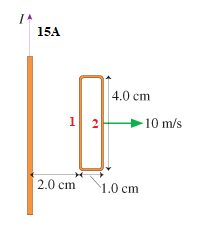# Problem: The rectangular loop in the figure has 2.1×10−2 ohm resistance. What is the induced current in the loop at this instant?

###### FREE Expert Solution

In this problem, the loop is moving. Thus, we are dealing with motional emf.

Emf:

$\overline{){\mathbf{\epsilon }}{\mathbf{=}}{\mathbf{B}}{\mathbf{l}}{\mathbf{v}}{\mathbf{s}}{\mathbf{i}}{\mathbf{n}}{\mathbf{\theta }}}$

The magnetic field due to a current-carrying conductor:

$\overline{){\mathbf{B}}{\mathbf{=}}\frac{{\mathbf{\mu }}_{\mathbf{0}}\mathbf{i}}{\mathbf{2}\mathbf{\pi }\mathbf{r}}}$

82% (86 ratings)###### Problem Details

The rectangular loop in the figure has 2.1×10−2 ohm resistance. What is the induced current in the loop at this instant?